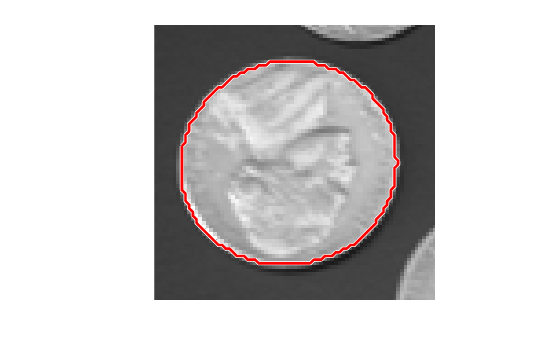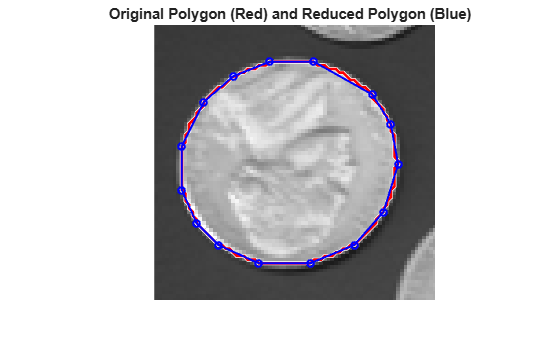# reducepoly

Reduce density of points in ROI using Ramer–Douglas–Peucker algorithm

## Syntax

``P_reduced = reducepoly(P)``
``P_reduced = reducepoly(P,tolerance)``

## Description

example

````P_reduced = reducepoly(P)` reduces the density of points in array `P`. The `reducepoly` function uses the Ramer-Douglas-Peucker line simplification algorithm, removing points along straight lines and leaving only knickpoints (points where the line curves).```
````P_reduced = reducepoly(P,tolerance)` reduces the density of points in array `P`, where `tolerance` specifies how much a point can deviate from a straight line.```

## Examples

collapse all

Read an image into the workspace.

```I = imread('coins.png'); imshow(I)```Convert the image from grayscale to binary.

`bw = imbinarize(I);`

Obtain the boundaries of all the coins in the binary image.

`[B,L] = bwboundaries(bw,'noholes');`

Select the boundary of the first detected coin.

```coinNumber = 1; boundary = B{coinNumber};```

Plot the boundary for the first detected coin over the original image.

```hold on visboundaries({boundary}) xlim([min(boundary(:,2))-10 max(boundary(:,2))+10]) ylim([min(boundary(:,1))-10 max(boundary(:,1))+10]) hold off```Use `reducepoly` to reduce the number of points defining the coin boundary. Return a smaller number of points by increasing the tolerance from the default value of `0.001`.

```tolerance = 0.02; p_reduced = reducepoly(boundary,tolerance);```

To see how well the reduced polygon matches the original polygon, plot the reduced polygon vertices over the image.

```line(p_reduced(:,2),p_reduced(:,1), ... 'color','b','linestyle','-','linewidth',1.5,... 'marker','o','markersize',5); title('Original Polygon (Red) and Reduced Polygon (Blue)');```## Input Arguments

collapse all

Points to be reduced, specified as an n-by-2 numeric matrix of the form `[x1 y1; ...; xn yn]`. Each row in the array defines a vertex in an ROI shape, such as a polyline, polygon, or freehand.

For example, you can draw a freehand ROI by using the `drawfreehand` function. Then, get the ROI vertices from the `Position` property of the freehand ROI object.

```roi = drawfreehand; P = roi.Position;```

Data Types: `single` | `double` | `int8` | `int16` | `int32` | `int64` | `uint8` | `uint16` | `uint32` | `uint64`

Sensitivity of the reduction algorithm, specified as a numeric scalar in the range [0, 1]. Increasing the tolerance increases the number of points removed. A tolerance value of `0` has a minimum reduction in points. A tolerance value of `1` results in maximum reduction in points, leaving only the end points of the line.

Data Types: `single` | `double` | `int8` | `int16` | `int32` | `int64` | `uint8` | `uint16` | `uint32` | `uint64`

## Output Arguments

collapse all

Reduced data set, returned as an m-by-2 numeric matrix. The number of reduced points is usually smaller than the number of original points in `P`.

Data Types: `double`

## Algorithms

The Ramer-Douglas-Peucker line simplification algorithm recursively subdivides a shape looking to replace a run of points with a straight line. The algorithm checks that no point in the run deviates from the straight line by more than the value specified by `tolerance`.